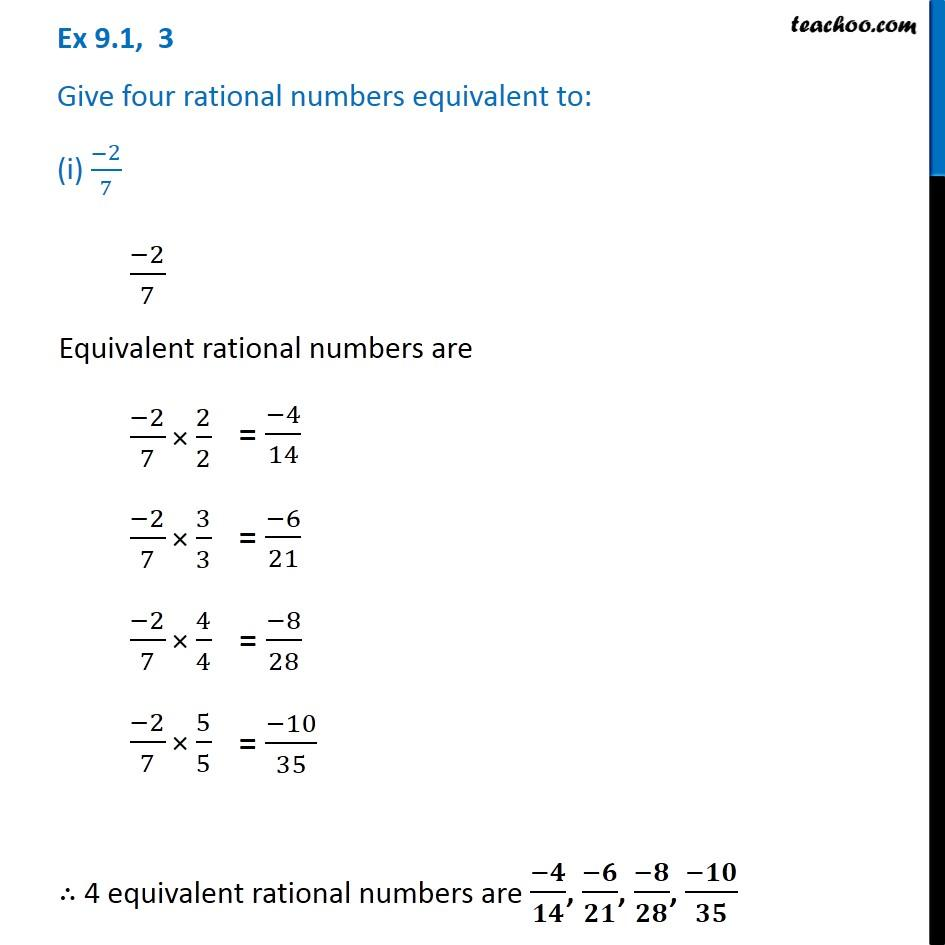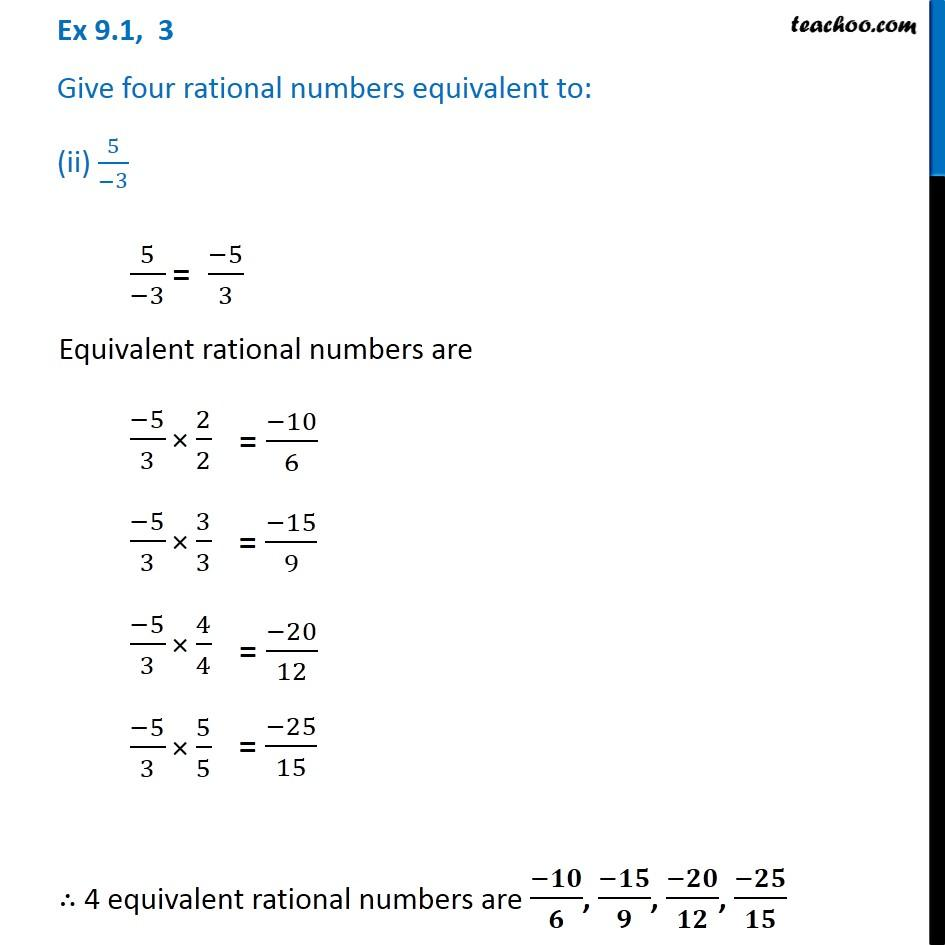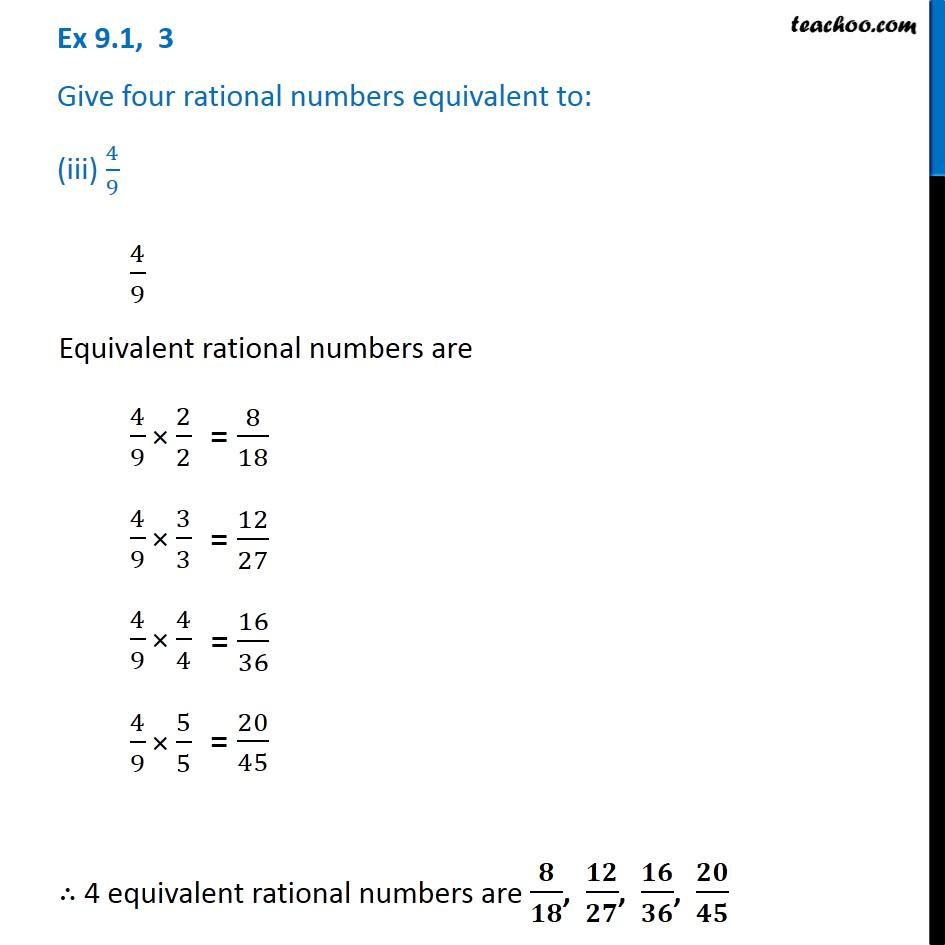Subscribe to our Youtube Channel - https://you.tube/teachoo

1. Chapter 9 Class 7 Rational Numbers
2. Concept wise
3. Equivalent Rational Numbers

Transcript

Ex 9.1, 3 Give four rational numbers equivalent to: (i) (-2)/7 (−2)/7 Equivalent rational numbers are (−2)/7 × 2/2 (−2)/7 × 3/3 (−2)/7 × 4/4 (−2)/7 × 5/5 ∴ 4 equivalent rational numbers are (−𝟒)/𝟏𝟒, (−𝟔)/𝟐𝟏, (−𝟖)/𝟐𝟖, (−𝟏𝟎)/𝟑𝟓 Ex 9.1, 3 Give four rational numbers equivalent to: (ii) 5/(−3) 5/(−3) = (−5)/3 Equivalent rational numbers are (−5)/3 × 2/2 (−5)/3 × 3/3 (−5)/3 × 4/4 (−5)/3 × 5/5 ∴ 4 equivalent rational numbers are (−𝟏𝟎)/𝟔, (−𝟏𝟓)/𝟗, (−𝟐𝟎)/𝟏𝟐, (−𝟐𝟓)/𝟏𝟓 Ex 9.1, 3 Give four rational numbers equivalent to: (iii) 4/9 4/9 Equivalent rational numbers are 4/9 × 2/2 4/9 × 3/3 4/9 × 4/4 4/9 × 5/5 ∴ 4 equivalent rational numbers are 𝟖/𝟏𝟖, 𝟏𝟐/𝟐𝟕, 𝟏𝟔/𝟑𝟔, 𝟐𝟎/𝟒𝟓

Equivalent Rational Numbers